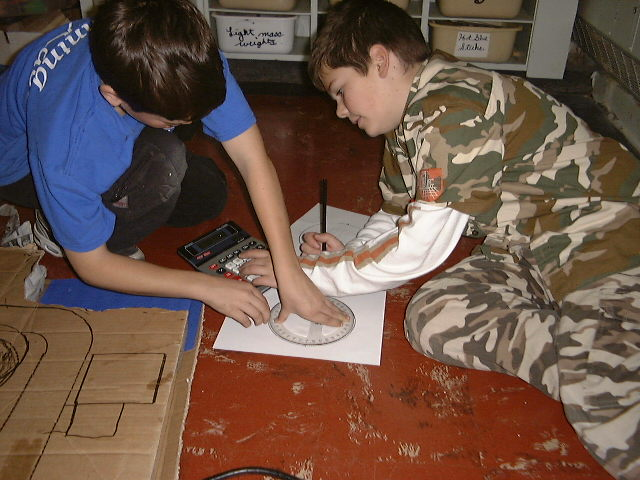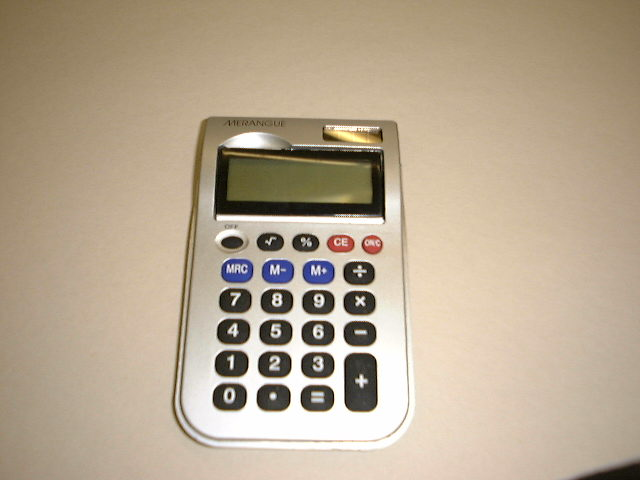The CalculatorThese two students are using a calculator to calculate the number of degrees that are required to divide the circle protractor into three equal parts.

These three points, when joined together, will form into an equilateral triangle. The equilateral triangle will be used as a template to make a tetrahedron.

This simple calculator is a good example of a calculator we used in my classroom. Each student was required to have and organize their calculator.

Calculators helped me teach math operations. A calculator promotes thinking and reasoning skills when solving problems.

When students were reading in the class as a group or individually they were required to have their calculator beside them. At any time, I could ask them the fraction, decimal equivalent or percentage of progress they were making toward completing their book.

My students used a calculator for a variety of other mathematical purposes. The calculator made solving math problems an enjoyable experience.